# Generating ready-to-analyze datasets with GRD

The package superb includes the function GRD(). This function is used to easily generate random data sets. With a few options, it is possible to obtain data from any design, with any effects. This function, first created for SPSS Harding & Cousineau (2015) was exported to R (Calderini & Harding, 2019). A brief report shows one possible use in the class for teaching statistics to undergrads (Cousineau, 2020).

This vignette illustrate some of its use.

## Simplest specification

The simplest use relies on the default value:

dta <- GRD()
head(dta)
##   id          DV
## 1  1  0.60482289
## 2  2 -0.12343132
## 3  3  0.47309422
## 4  4  0.01490582
## 5  5 -1.09430858
## 6  6  0.60422817

By default, one hundred scores are generated from a normal distribution with mean 0 and standard deviation of 1. In other words, it generate 100 z scores. The dependent variable, the last column in the dataframe that will be generated is called by default DV. The first column is an “id” column containing a number identifying each simulated participant. To change the dependent variable’s name, use

dta <- GRD( RenameDV = "score" )

## Data from a design with between-subject factors and within-subject factors.

To add various groups to the dataset, use the argument BSFactors, as in

dta <- GRD( BSFactors = 'Group(3)')

There will be 100 random z scores in each of three groups, for a total of 300 data. The group number will be given in an additional column, here called Group. A factorial design can be generated with more than one factors, such as

dta <- GRD( BSFactors = c('Surgery(2)', 'Therapy(3)') )

which will results in 2 $$\times$$ 3, that is, 6 different groups, crossing all the levels of Surgery (1 and 2) and all the levels of Therapy (1, 2 and 3). The levels can receive names rather than number, as in

dta <- GRD(
BSFactors = c('Surgery(yes, no)', 'Therapy(CBT,Control,Exercise)')
)
unique(dta$Surgery) ##  "yes" "no" unique(dta$Therapy)
##  "CBT"      "Control"  "Exercise"

Finally, within-subject factors can also be given, as in

dta <- GRD(
BSFactors = c('Surgery(yes,no)', 'Therapy(CBT, Control,Exercise)'),
WSFactors = 'Contrast(C1,C2,C3)',
)

For within-subject designs, the repeated measures will appear in distinct columns (here “DV.C1”, “DV.C2”, and “DV.C3” ). This format is called wide format, meaning that the repeated measures are all on the same line for a given simulated participant.

## Deciding the sample sizes

The default is to generate 100 participants in each between-subject groups. This default can be changed with SubjectsPerGroup. The most straigthforward specification is, e.g., SubjectsPerGroup = 25 for 25 participants in each groups. Unequal group sizes can be specified with:

dta <- GRD(
BSFactors = "Therapy(3)",
SubjectsPerGroup = c(2, 5, 1)
)
dta
##   id Therapy         DV
## 1  1       1  0.7230224
## 2  2       1  0.3112748
## 3  3       2  0.8723501
## 4  4       2  0.3690768
## 5  5       2 -0.4116056
## 6  6       2  0.3542911
## 7  7       2 -0.6744981
## 8  8       3  1.2693458

## Choosing the population distribution

To sample random data, it is necessary to specify a theoretical population distribution. The default is to use a normal distribution (the famous “bell-shaped” curve). That population has a grand mean (GM, $$\mu$$) given by the element mean and standard deviation ($$\sigma$$) given by the element stddev. These can be redefined using the argument Population with a list of the relevant elements. In the following example, IQ are being simulated with :

dta <- GRD(
RenameDV = "IQ",
Population=list(mean=100,stddev=15)
)
hist(dta$IQ)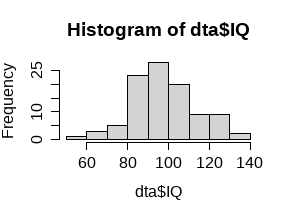(increase the number of participants using SubjectsPerGroup to say 10,000, and the bell-shape curve will be evident!). Internally, the above call to GRD() will use rnorm to generate the scores, passing along for the mean parameter the grand mean (internally called GM) and for the standard deviation parameter the provided standard deviation (internally called STDDEV). This can be explicitly stated using the element scores as in: dta <- GRD( BSFactors = "Group(2)", Population = list( mean = 100, # this set GM to 100 stddev = 15, # this set STDDEV to 15 scores = "rnorm(1, mean = GM, sd = STDDEV )" ) ) Using scores, it is possible to alter the parameters, for example, have a mean proportional to the group number, or the standard deviation proportional to the group number, as in: dta <- GRD( BSFactors = "Group(2)", Population = list( mean = 100, # this set GM to 100 stddev = 15, # this set STDDEV to 15 scores = "rnorm(1, mean = GM, sd = Group * STDDEV )" ) ) superbPlot(dta, BSFactors = "Group", variables = "DV", plotStyle = "pointjitterviolin" )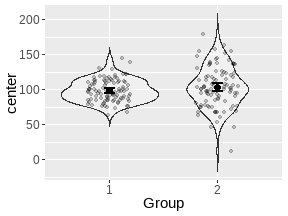Any valid R instruction could be placed in the scores arguments, such as scores = "rnorm(1, mean = GM, sd = ifelse(Group==1,10,50) )" to select the standard deviation according to Group or scores = "1" to generate constants. Other theoretical distributions can also be chosen, as in: dta <- GRD(SubjectsPerGroup = 5000, RenameDV = "RT", Population=list( scores = "rweibull(1, shape=2, scale=40)+250" ) ) hist(dta$RT,breaks=seq(250,425,by=5))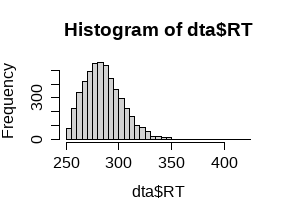## Getting effects on one or some of the factors

It is possible to generate non-null effects on the factors using the argument Effects. Effects can be slope(x) (an increase of x points for each level of the factor), extent(x) (a total increase of x over all the levels), custom(x, y, etc) for an effect of x point for the first level of the factor, y point for the second, etc.

Here is a slope effect:

dta <- GRD(
BSFactors = 'Therapy(CBT, Control, Exercise)',
WSFactors = 'Contrast(3)',
SubjectsPerGroup = 1000,
Effects = list('Contrast' = slope(2))
)
superbPlot(dta,
BSFactors = "Therapy", WSFactors = "Contrast(3)",
variables = c("DV.1","DV.2","DV.3"),
plotStyle = "line" )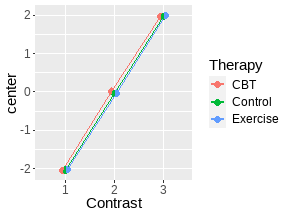Effects can also be any R code manipulating the factors, using Rexpression. One example:

dta <- GRD(
BSFactors = 'Therapy(CBT,Control,Exercise)',
WSFactors = 'Contrast(3) ',
SubjectsPerGroup = 1000,
Effects = list(
"code1"=Rexpression("if (Therapy =='CBT'){-1} else {0}"),
"code2"=Rexpression("if (Contrast ==3) {+1} else {0}")
)
)
superbPlot(dta,
BSFactors = "Therapy", WSFactors = "Contrast(3)",
variables = c("DV.1","DV.2","DV.3"),
plotStyle = "line" )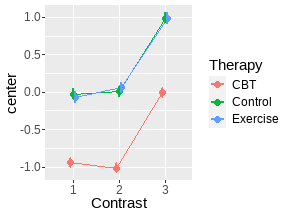Repeated measures can also be generated from a multivariate normal distribution with a correlation rho, with, e.g.,

dta <- GRD(
WSFactors = 'Difficulty(1, 2)',
SubjectsPerGroup = 1000,
Population=list(mean = 0,stddev = 20, rho = 0.5)
)
plot(dta$DV.1, dta$DV.2)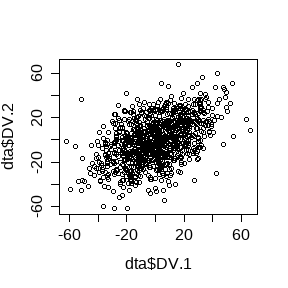In the case of a multivariate normal distribution, the parameters for the mean and the standard deviations can be vectors of length equal to the number of repeated measures. However, covariances are constants.

dta <- GRD(
WSFactors = 'Difficulty(1, 2)',
SubjectsPerGroup = 1000,
Population=list(mean = c(10,2),stddev= c(1,0.2),rho =-0.85)
)
plot(dta$DV.1, dta$DV.2)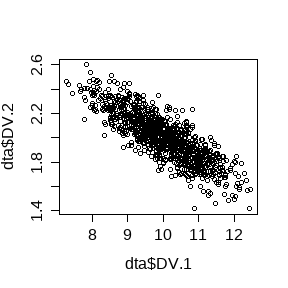Contaminants can be inserted in the simulated data using Contaminant. This argument works exactly like Population except for the additional option proportion which indicates the proportion of contaminants in the samples:

dta <- GRD(SubjectsPerGroup = 5000,
Population= list( mean=100, stddev = 15 ),
Contaminant=list( mean=200, stddev = 15, proportion = 0.10 )
)
hist(dta$DV,breaks=seq(-25,300,by=2.5))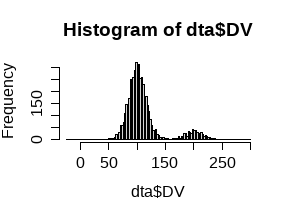Contaminants can be normally distributed (as above) or come from any theoretical distribution which can be simulated in R: dta <- GRD(SubjectsPerGroup = 10000, Population=list( mean=100, stddev = 15 ), Contaminant=list( proportion = 0.10, scores="rweibull(1,shape=1.5, scale=30)+1.5*GM") ) hist(dta$DV,breaks=seq(0,365,by=2.5))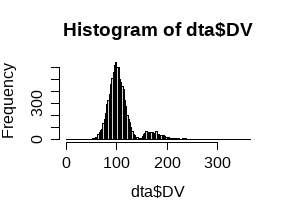Finally, contaminants can be used to add missing data (missing completely at random) with:

dta <- GRD( BSFactors="grp(2)",
WSFactors = "Moment (2)",
SubjectsPerGroup = 1000,
Effects = list("grp" = slope(100) ),
Population=list(mean=0,stddev=20,rho= -0.85),
Contaminant=list(scores = "NA", proportion=0.2)
)

## In summary

GRD() is a convenient function to generate about any sorts of data sets with any form of effects. The data can simulate any factorial designs involving between-subject designs, repeated-measure designs, and multivariate data.

One use if of course in the classroom: students can test their skill by generating random data sets and run statistical procedures. To illustrate type-I errors, it become then easy to generate data with no effect whatsoever and ask the students who obtain a rejection decision to raise their hand.